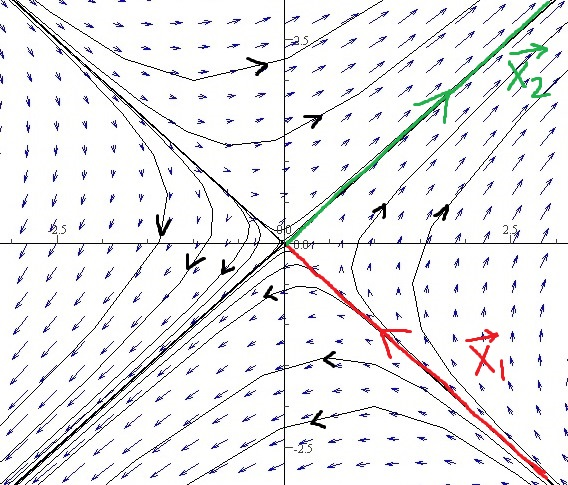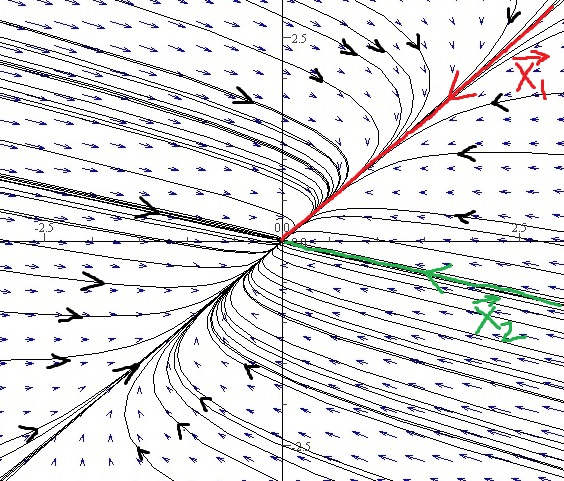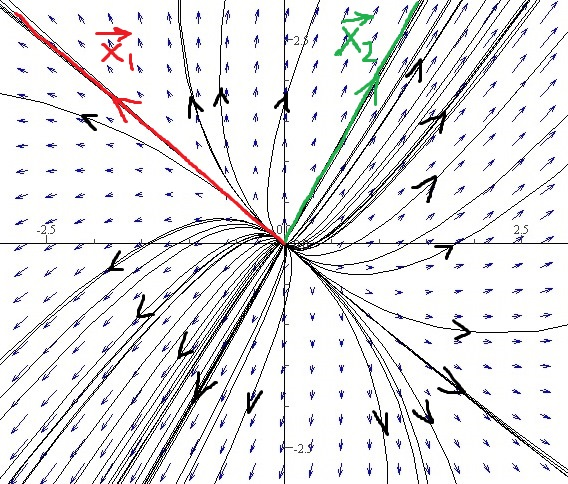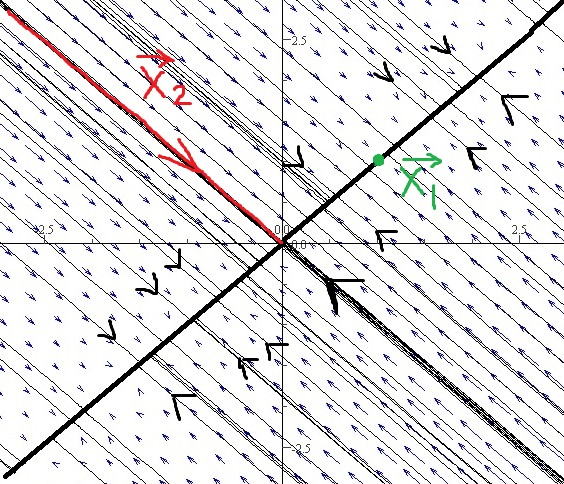DiffEq Home

## Equilibrium Solutions and Phase Portraits

Consider the following system of $$n$$ linear ODEs: $\overrightarrow{x}'=A\overrightarrow{x}, \;\;\;\;(53)$ where $$\overrightarrow{x}=[x_1,\; x_2,\cdots,\; x_n]^T$$. $$\overrightarrow{x}$$ is called an equilibrium solution of (53) if $$A\overrightarrow{x}=\overrightarrow{0}$$. Obviously $$\overrightarrow{x}=\overrightarrow{0}$$ is always an equilibrium solution of (53). Note that if $$A$$ is invertible (i.e., $$\det A\neq 0$$ or, all eigenvalues of $$A$$ are nonzero), then $$A\overrightarrow{x}=\overrightarrow{0}\implies \overrightarrow{x}=A^{-1}\overrightarrow{0}=\overrightarrow{0}$$ and $$\overrightarrow{x}=\overrightarrow{0}$$ is the only equilibrium solution of (53).

Direction field/ tangent field: A solution curve or a trajectory of (53) can be visualized by plotting direction field of tangent vectors to solutions of (53). $$\overrightarrow{x}'=A\overrightarrow{x}$$ is the tangent vector of a solution curve at $$\overrightarrow{x}\in \mathbb R^n$$.

We mostly focus on the case $$n=2$$ and plot trajectories on the $$x_1x_2$$-plane which is called the phase plane. A sketch of trajectories on the phase plane is called a phase portrait.

Example. $$\overrightarrow{x}'=\left[\begin{array}{rr} 1&2\\2&1\end{array} \right] \overrightarrow{x}$$.

1. Draw direction field. Use the points: $$(0,0),(\pm1,0),(0,\pm 1),(\pm 1,\pm 1)$$.

2. Draw the phase portrait.

Solution. (a) At $$\overrightarrow{x}=\left[\begin{array}{r}0\\0\end{array} \right],\; \overrightarrow{x}'=\left[\begin{array}{rr} 1&2\\2&1\end{array} \right] \left[\begin{array}{r}0\\0\end{array} \right]= \left[\begin{array}{r}0\\0\end{array} \right].$$
At $$\overrightarrow{x}=\left[\begin{array}{r}\pm 1\\0\end{array} \right],\; \overrightarrow{x}'=\left[\begin{array}{rr} 1&2\\2&1\end{array} \right] \left[\begin{array}{r}\pm 1\\0\end{array} \right]= \pm \left[\begin{array}{r}1\\2\end{array} \right].$$
At $$\overrightarrow{x}=\left[\begin{array}{r}0\\ \pm 1\end{array} \right],\; \overrightarrow{x}'=\left[\begin{array}{rr} 1&2\\2&1\end{array} \right] \left[\begin{array}{r}0\\ \pm 1\end{array} \right]= \pm \left[\begin{array}{r}2\\1\end{array} \right].$$
At $$\overrightarrow{x}=\pm \left[\begin{array}{r}1\\1\end{array} \right],\; \overrightarrow{x}'=\pm \left[\begin{array}{rr} 1&2\\2&1\end{array} \right] \left[\begin{array}{r}1\\ 1\end{array} \right]= \pm \left[\begin{array}{r}3\\3\end{array} \right].$$
At $$\overrightarrow{x}=\pm \left[\begin{array}{r}1\\-1\end{array} \right],\; \overrightarrow{x}'=\pm \left[\begin{array}{rr} 1&2\\2&1\end{array} \right] \left[\begin{array}{r}1\\ -1\end{array} \right]= \pm \left[\begin{array}{r}-1\\1\end{array} \right].$$ Now draw the tangent vector $$A\overrightarrow{x}$$ at each $$\overrightarrow{x}$$.

(b) The eigenvalues of the coefficient matrix are $$-1$$ and $$3$$ with corresponding eigenvectors $$\left[\begin{array}{r}1\\-1\end{array} \right]$$ and $$\left[\begin{array}{r}1\\1\end{array} \right]$$ respectively (show all the steps). So the general solution is $\overrightarrow{x}(t)= c_1e^{-t}\left[\begin{array}{r}1\\-1\end{array} \right] +c_2e^{3t}\left[\begin{array}{r}1\\1\end{array} \right].$ When $$c_2=0$$, $$\overrightarrow{x}=c_1\overrightarrow{x_1}=c_1e^{-t}\left[\begin{array}{r}1\\-1\end{array} \right]$$. This gives the trajectory $$x_1=c_1e^{-t},\; x_2=-c_1e^{-t}$$, i.e., the line $$x_2=-x_1$$ (the line through the origin parallel to $$\overrightarrow{v_1}=\left[\begin{array}{r}1\\-1\end{array} \right]$$). This trajectory is in the quadrant 4 when $$c_1 > 0$$ and in the quadrant 2 when $$c_1 < 0$$. Note that $$\overrightarrow{x}=c_1\overrightarrow{x_1}=c_1e^{-t}\left[\begin{array}{r}1\\-1\end{array} \right]$$ is far from the origin for large negative $$t$$ and $$\overrightarrow{x}=c_1\overrightarrow{x_1}=c_1e^{-t}\left[\begin{array}{r}1\\-1\end{array} \right]\to \left[\begin{array}{r}0\\0\end{array} \right]$$ as $$t\to \infty$$.

When $$c_1=0$$, $$\overrightarrow{x}=c_2\overrightarrow{x_2}=c_2e^{3t}\left[\begin{array}{r}1\\1\end{array} \right]$$. This gives the trajectory $$x_1=c_2e^{3t},\; x_2=c_2e^{3t}$$, i.e., the line $$x_2=x_1$$ (the line through the origin parallel to $$\overrightarrow{v_2}=\left[\begin{array}{r}1\\1\end{array} \right]$$). This trajectory is in the quadrant 1 when $$c_2 > 0$$ and in the quadrant 3 when $$c_2 < 0$$. Note that $$\overrightarrow{x}=c_2\overrightarrow{x_2}=c_2e^{3t}\left[\begin{array}{r}1\\1\end{array} \right]$$ is far from the origin for large positive $$t$$ and $$\overrightarrow{x}=c_2\overrightarrow{x_2}=c_2e^{3t}\left[\begin{array}{r}1\\1\end{array} \right]\to \left[\begin{array}{r}0\\0\end{array} \right]$$ as $$t\to -\infty$$.Note:

1. For these kind of trajectories (when eigenvalues are real of opposite signs) in the preceding example, the origin is called a saddle point which is unstable as trajectories move away from the origin as $$t$$ increases.

2. When eigenvalues are distinct real of the same sign, the origin is called a node which is asymptotically stable when eigenvalues are negative (trajectories move toward the origin as $$t$$ increases) and unstable when eigenvalues are positive (trajectories move away from the origin as $$t$$ increases).

Example. $$\overrightarrow{x}'=\left[\begin{array}{rr} -5&4\\1&-2\end{array} \right] \overrightarrow{x}$$.

Solution. The general solution is $\overrightarrow{x}(t)= c_1e^{-t}\left[\begin{array}{r}1\\1\end{array} \right] +c_2e^{-6t}\left[\begin{array}{r}4\\-1\end{array} \right].$ $$c_2\overrightarrow{x_2}$$ will dominate $$c_1\overrightarrow{x_1}$$ for large negative $$t$$ (i.e., when far from the origin). $$c_1\overrightarrow{x_1}$$ will dominate $$c_2\overrightarrow{x_2}$$ for large positive $$t$$ (i.e., when near the origin). The origin is an asymptotically stable node.Example. $$\overrightarrow{x}'=\left[\begin{array}{rr} 3&1\\2&4\end{array} \right] \overrightarrow{x}$$.

Solution. The general solution is $\overrightarrow{x}(t)= c_1e^{2t}\left[\begin{array}{r}-1\\1\end{array} \right] +c_2e^{5t}\left[\begin{array}{r}1\\2\end{array} \right].$ The origin is an unstable node.Example. $$\overrightarrow{x}'=\left[\begin{array}{rr} -1&1\\1&-1\end{array} \right] \overrightarrow{x}$$.

Solution. The general solution is $\overrightarrow{x}(t)= c_1\left[\begin{array}{r}1\\1\end{array} \right] +c_2e^{-2t}\left[\begin{array}{r}-1\\1\end{array} \right].$ Each point on the line $$x_2=x_1$$ is an equilibrium. The origin is a degenerate node.Note. The origin is asymptotically stable when both eigenvalues are negative (trajectories move toward the origin as $$t$$ increases) and unstable when at least one eigenvalue is positive (trajectories move away from the origin as $$t$$ increases).

Last edited